# Maharashtra Board Class 6 Maths Solutions Chapter 2 Angles Practice Set 3

## Maharashtra State Board Class 6 Maths Solutions Chapter 2 Angles Practice Set 3

Question 1.
Use the proper geometrical instruments to construct the following angles. Use the compass and the ruler to bisect them:

1. 50°
2. 115°
3. 80°
4. 90°

Solution:Maharashtra Board Class 6 Maths Chapter 2 Angles Practice Set 3 Intext Questions and Activities

Question 1.
Construct an angle bisector to obtain an angle of 30°. (Textbook pg. no. 11)
Solution: .
In order to get a bisected angle of a given measure, the student has to draw the angle having twice the measurement of required bisected angle.

For getting measurement of 30° (for the bisected angle), one has to make an angle of 60° (i.e. 30° × 2).

Step 1:
Draw ∠ABC of 60°.Step 2:
Cut arcs on the rays BA and BC. Name these points as D and E respectively.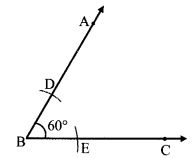Step 3:
Place the compass point on point D and draw an arc inside the angle.
Without changing the distance of the compass, place the compass point on point E and cut the previous arc. Name the point of intersection as O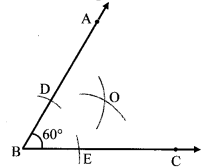Step 4:
Draw ray BO.
Ray BO is the angle bisector of ∠ABC.
i.e. m∠ABO = m∠CBO = 30°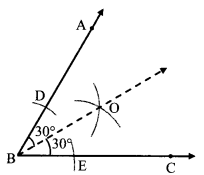Question 2.
Construct an angle bisector to draw an angle of 45°. (Textbook pg. no. 11)
Solution:
For getting measurement of 45° (for the bisected angle), one has to make an angle of 90° (i.e. 45° × 2).
Step 1:
Draw ∠PQR of 90°.Step 2:
Cut arcs on the rays QP and QR.
Name these points as M and N respectively.Step 3:
Place the compass point on point M and draw an arc inside the angle.
Without changing the distance of the compass, place the compass point on point N and cut the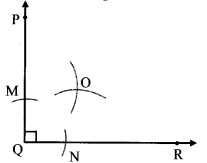Step 4:
Draw ray QO.
Ray QO is the angle bisector of ∠PQR.
i.e. m∠PQO = m∠RQO = 45°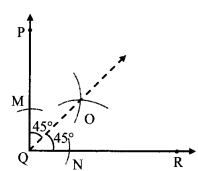Question 3.
Ask three or more children to stand in a straight line. Take two long ropes. Let the child in the middle hold one end of each rope. With the help of the ropes, make the children on either side stand along a straight line. Tell them to move so as to form an acute angle, a right angle, an obtuse angle, a straight angle, a reflex angle and a full or complete angle in turn. Keeping the rope stretched will help to ensure that the children form straight lines. (Textbook pg. no. 6)
Solution: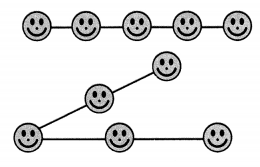Question 4.
Look at the pictures below and identify the different types of angles. (Textbook pg. no. 8)Solution:
i. Complete angle
ii. Reflex and Acute angle
iii. Acute and Obtuse angle